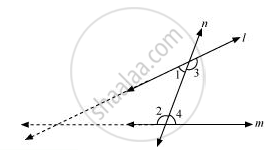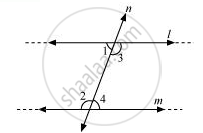# Does Euclid’s fifth postulate imply the existence of parallel lines? Explain. - Mathematics

Does Euclid’s fifth postulate imply the existence of parallel lines? Explain.

#### Solution

Yes.

According to Euclid’s 5th postulate, when n line falls on l and m and if

∠1 + ∠2 < 180°, then ∠3 + ∠4 > 180°, producing line l and m further will meet in the side of ∠1 and ∠2 which is less than 180°.If ∠1 + ∠2 = 180°, then ∠3 + ∠4 = 180°The lines l and m neither meet at the side of ∠1 and ∠2 nor at the side of ∠3 and ∠4. This means that the lines l and m will never intersect each other. Therefore, it can be said that the lines are parallel.

Concept: Equivalent Versions of Euclid’S Fifth Postulate
Is there an error in this question or solution?
Chapter 5: Introduction to Euclid's Geometry - Exercise 5.2 [Page 88]

#### APPEARS IN

NCERT Class 9 Maths
Chapter 5 Introduction to Euclid's Geometry
Exercise 5.2 | Q 2 | Page 88

Share